#1 Vancouver Flooring Installers And Suppliers Since 1999

#1 Vancouver Flooring Company Since 1999. Ask For Your Free Estimates Today!

Are you planning to renovate your room and change the flooring material? Or maybe you want to install a wall-to-wall carpet. If so, then one of the things that you should know is the square feet measurement of the room. Although, it’s not as easy as it sounds. Some places are perfect square or rectangle, but some have odd shapes. Still, you need to measure even the smallest part of a room, in order come up with exact area calculation. It takes time and patience. But knowing how to measure the square feet (Area) of your room accurately is very important.
How so? It is essential so you can buy the correct number of tiles or yards of carpet, and not waste any money. If you want to know how many boxes of wooden parquet or ceramic tiles you should buy, calculate how many square feet of materials there is per box. Then, measure the rooms’ total square footage.

## How to measure the square feet of your room

The formula to get a room’s square footage is simple. Room length multiplied by the room width (L x W). However, it gets a little more complicated for rooms which are not perfect in shape. We listed some room examples along with the procedure of measuring their total square feet. Find which room shape is most similar to yours, then use the corresponding method to measure the square feet of your room.

## How to measure the square feet of a perfectly rectangular room.

This one is straightforward. To measure a simple rectangular room’s square feet, just multiply the room length by the room width. Make sure that you measure from the inside of the room and that you do the measurement as accurately as possible. Below is the equation to measure the square feet of a simple rectangular room:

#### Length x Width = Room Square Feet

As an example, we will find the square feet of a room with a length of 20 ft. and a width of 15 ft.

#### 20 ft. x 15 ft. = 300 square feet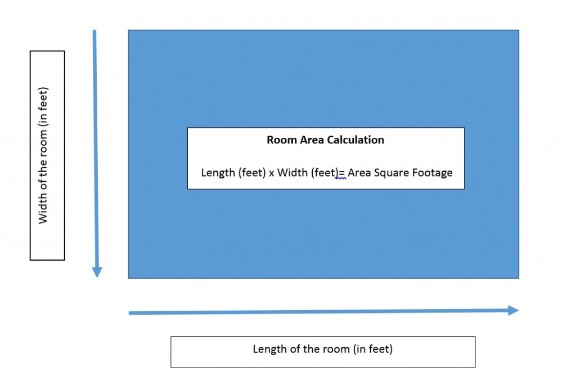## How to measure the square feet of a room with closets.

This is where it gets tricky. If you have closets in your room and you plan to install floorings on them also, then you need to combine the square feet of the cabinets with the room’s measurement. Here is the calculation method:

• Separate the room into different areas – You can tag the areas as “Room”, “Closet A”, “Closet B”, and so on depending on the number of closets.
• Calculate the square feet of each area using the basic formula:

#### Length x Width = Area Square Feet

• For example, the room in step 1 (300 square feet) has two closets, Closet A and Closet B. Closet A has a length of 10 ft. and width of 2 ft. Closet B has a length of 5 ft. and width of 2 ft. Compute for the square feet for each of the two closets:

#### Length x Width = Square Feet (Closet B) 5 ft. x 2 ft. = 10 Square Feet (Closet B)

• To measure the total square feet, add the square feet of the room, plus the that of Closet A and Closet B:

#### 300 sq.-ft. + 20 sq.-ft. + 10 sq.-ft. = 330 Square Feet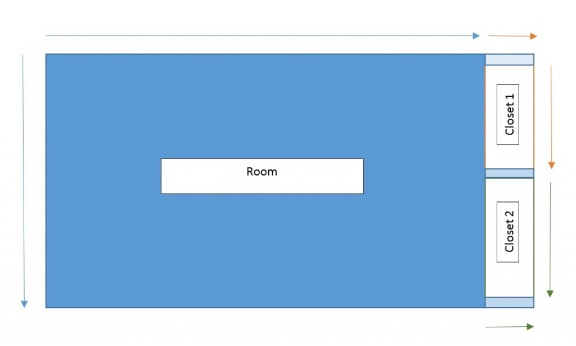## How to measure the square feet of a room with the odd shape.

Most people who find it hard to measure the square feet of an oddly spaced room try to do the entire space at one time. This is a very wrong move. Although it is not very often that you see a place with many closets, nooks and crannies, and complex shapes, it should never be a problem measuring its square feet. The solution is to just split the room into even rectangles, then calculate the square feet of each rectangle by the basic length x width formula. To calculate the total square feet of the oddly-shaped room, just add the square feet of each rectangle.

• Separate the room into different rectangles – You can tag the squares as “Area A”, “Area B”, and so on depending on the number of rectangles.
• Calculate the square feet of each rectangle using the basic formula:

#### Length x Width = Rectangle Square Feet

For example, the room in step 1 and 2(330 square feet) has three more areas connected to it, Area A, Area B and Area C. Area A has a length of 10 ft. and width of 5 ft. Area B has a length of 10 ft. and width of 10 ft. Finally, Area C has the length of 10 ft. and width of 7 ft. Compute for the square feet for each of the three areas:

#### Length x Width = Square Feet (Area C) 10 ft. x 7 ft. = 70 Square Feet (Area C)

• To measure the total square feet, add the square feet of the room, plus the that of Area A, Area B and Area C:

## Remember to Include Possible Waste to Your Calculation

Don’t forget to include 5-10% extra when calculating the total square feet of your flooring materials. This is the possible waste due to damage or errors when installing the tiles or carpet. It’s always better to have a few extras ready. To calculate the 5% waste and the total square feet of flooring materials, follow these two easy formulas:

1. Total Room Square Feet x 0.05 = Waste Materials
2. Total Room Square Feet + Waste Materials Square Feet = Total square feet of flooring materials

Let’s take the 550 Square Feet room as an example:

#### 550 sq.-ft + 27.5 sq.-ft. = 577.5 Total square feet of flooring materials

Now you know how to measure the square feet of your room and the total square feet of flooring materials. Just remember when purchasing your flooring materials, round up or down the full square feet based on the measurement of each tile or parquet. If you want to know more about floorings and square feet calculations, you can call us, and we will be happy to help you.

##### Have wood flooring questions? BC BEST FLOORING® is by your side. Let us help!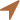Our Location

1641 Lonsdale Ave., Suite #1016, North Vancouver, BC, V7M2J5 (for mail only)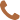Call Us

(604) 373 3377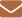### Here when you need us

Send us your questions and requests.

Fill out the form below to send us any questions, requests, or general comments. We'd love to hear from you.